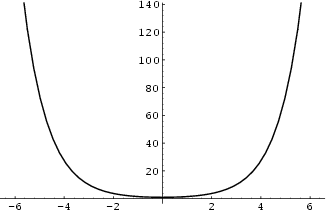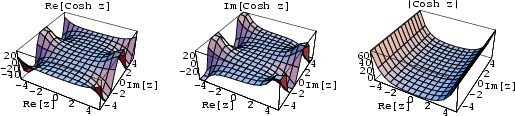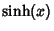## Hyperbolic CosineThe hyperbolic cosine is defined asThis function describes the shape of a hanging cable, known as the Catenary.

See also Bipolar Coordinates, Bipolar Cylindrical Coordinates, Bispherical Coordinates, Catenary, Catenoid, Chi, Conical Function, Correlation Coefficient--Gaussian Bivariate Distribution, Cosine, Cubic Equation, de Moivre's Identity, Elliptic Cylindrical Coordinates, Elsasser Function, Fibonacci Hyperbolic Cosine, Fibonacci Hyperbolic Sine, Hyperbolic Geometry, Hyperbolic Lemniscate Function, Hyperbolic Sine, Hyperbolic Secant, Hyperbolic Tangent, Inversive Distance, Laplace's Equation--Bipolar Coordinates, Laplace's Equation--Bispherical Coordinates, Laplace's Equation--Toroidal Coordinates, Lemniscate Function, Lorentz Group, Mathieu Differential Equation, Mehler's Bessel Function Formula, Mercator Projection, Modified Bessel Function of the First Kind, Oblate Spheroidal Coordinates, Prolate Spheroidal Coordinates, Pseudosphere, Ramanujan Cos/Cosh Identity, Sine-Gordon Equation, Surface of Revolution, Toroidal Coordinates

References

Abramowitz, M. and Stegun, C. A. (Eds.). Hyperbolic Functions.'' §4.5 in Handbook of Mathematical Functions with Formulas, Graphs, and Mathematical Tables, 9th printing. New York: Dover, pp. 83-86, 1972.

Spanier, J. and Oldham, K. B. The Hyperbolic Sineand CosineFunctions.'' Ch. 28 in An Atlas of Functions. Washington, DC: Hemisphere, pp. 263-271, 1987.